Formal DefinitionsNext: Safety of Expressions Up: The Tuple Relational Previous: Example Queries

## Formal Definitions

1. A tuple relational calculus expression is of the form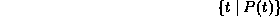where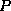is a formula.

Several tuple variables may appear in a formula.

2. A tuple variable is said to be a free variable unless it is quantified by aor a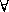. Then it is said to be a bound variable.

3. A formula is built of atoms. An atom is one of the following forms:
•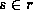, whereis a tuple variable, and r is a relation (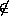is not allowed).
•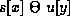, whereandare tuple variables, andandare attributes, and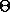is a comparison operator (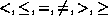).

•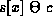, whereis a constant in the domain of attribute.
4. Formulae are built up from atoms using the following rules:
• An atom is a formula.
• Ifis a formula, then so are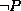and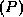.
• If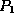and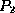are formulae, then so are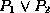,and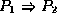.
• If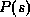is a formula containing a free tuple variable, then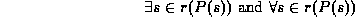are formulae also.

5. Note some equivalences:
•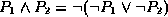•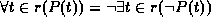•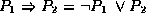Page created and maintained by Osmar R. Zaï ane
Last Update: Wed Sep 20 15:45:57 PDT 1995